# The Metagyrate Diminished Rhombicosidodecahedron

The metagyrate diminished rhombicosidodecahedron is the 78th Johnson solid, or J78 for short. It has 52 faces (1 decagon, 11 pentagons, 25 squares, and 15 triangles), 105 edges, and 55 vertices. It is obtained by cutting off a pentagonal cupola segment from the rhombicosidodecahedron and gyrating a pentagonal cupola segment that isn't directly opposite the decagonal face.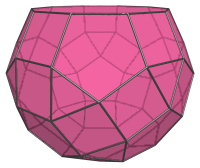The metagyrate diminished rhombicosidodecahedron closely resembles the diminished rhombicosidodecahedron (J76), but can be distinguished from the latter by 5 telltale pairs of adjacent squares, which are not present in J76: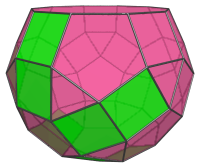These pairs of adjacent squares occur because of the gyrated orientation of the pentagonal cupola segment highlighted below: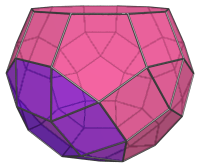## Projections

Here are some views of the metagyrate diminished rhombicosidodecahedron from various angles:

Projection Description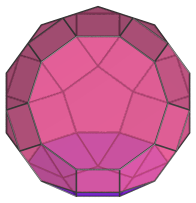Top view.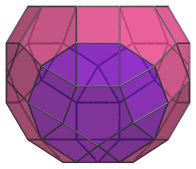Front view.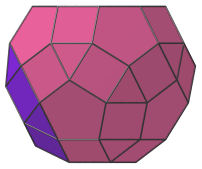Side view.

## Coordinates

The Cartesian coordinates of the metagyrate diminished rhombicosidodecahedron with edge length 2 are:

• (0, ±(2+φ), ±φ2)
• (±(2+φ), ±φ2, 0)
• (±φ2, 0, −(2+φ))
• (±φ2, ±2φ, ±φ)
• (±φ, ±φ2, ±2φ)
• (±2φ, ±φ, −φ2)
• (±1, ±1, ±(1+2φ))
• (±1, ±(1+2φ), ±1)
• (±(1+2φ), ±1, −1)
• (−(8φ+1)/5, ±1, (6φ+7)/5)
• (−(8φ+6)/5, ±φ, (φ+7)/5)
• (−(13φ+1)/5, 0, (φ+2)/5)

where φ=(1+√5)/2 is the Golden Ratio.

Last updated 17 May 2019.